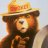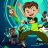# Why the watchlist column in not reflecting proper value?

K

#### kaflesu

##### New member
Hi all,

I am trying to build a watchlist scanner. My condition is that
1. if 50MA crosses the 200MA and the difference is within 1 to 3 % then pass 1
2. if 50MA crosses the 200MA and the difference is within more than 3 % then pass 2
3. if 50 MA is an uptrend and the % difference is less than 2% then 3
4. if 50 Ma is in uptrend than 4 else 5.

Question: Will I be able to choose a different time frame for the watchlist? When I select the Min, I get the error saying the secondary needs to be greater than the primary. In the graph, it is working perfectly fine with different time frames.

Code:
``````declare lower;

input length1 = 50;
input length2 = 200;
input price = close;
input averageType = AverageType.SIMPLE;

# plot the RSI Moving Average
def da1 = MovingAverage(averageType, close, length1);
def da2 = MovingAverage(averageType, close, length1);

def db1 = MovingAverage(averageType, close, length2);
def db2 = MovingAverage(averageType, close, length2);

def uptrend = (da1 - db1) >= (da2 - db2);
def downtrend = (da1 - db1) < (da2 - db2);
def whr = 100 * (da1 - db1) / da1;

plot signal =
if uptrend and whr > 1 and whr < 3 then 1
else if (uptrend and whr >= 3) then 2
else if (uptrend) and (whr > -2 )   then  3
else if (uptrend) then 4
else 5;

AssignBackgroundCOlor(if signal == 1 then Color.Green
else if signal== 2 then Color.Dark_Green
else if signal == 3 then Color.Orange
else if signal==4 then Color.yellow
else Color.Dark_Red);``````How do I convert this ATR% chart study into a scanner watchlist column? Questions 4Gap Info Watchlist Column for ThinkorSwim Custom 12